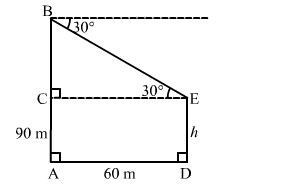# The horizontal distance between two towers is 60 metres.`
Question:

The horizontal distance between two towers is 60 metres. The angle of depression of the top of the first tower when seen from the top of the second tower is 30°. If the height of the second tower is 90 metres, find the height of the first tower $[$ Use $\sqrt{3}=1.732]$

Solution:

Let $D E$ be the first tower and $A B$ be the second tower.

Now, $A B=90 \mathrm{~m}$ and $A D=60 \mathrm{~m}$ such that $C E=60 \mathrm{~m}$ and $\angle B E C=30^{\circ}$.

Let $D E=h \mathrm{~m}$ such that $A C=h \mathrm{~m}$ and $B C=(90-h) \mathrm{m}$.In the right $\Delta B C E$, we have:

$\frac{B C}{C E}=\tan 30^{\circ}=\frac{1}{\sqrt{3}}$

$\Rightarrow \frac{(90-h)}{60}=\frac{1}{\sqrt{3}}$

$\Rightarrow(90-h) \sqrt{3}=60$

$\Rightarrow h \sqrt{3}=90 \sqrt{3}-60$

$\Rightarrow h=90-\frac{60}{\sqrt{3}}=90-34.64=55.36 \mathrm{~m}$

$\therefore$ Height of the first tower $=D E=h=55.36 \mathrm{~m}$